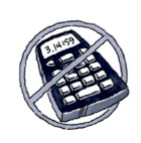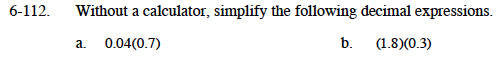### Home > MC1 > Chapter 6 > Lesson 6.2.6 > Problem6-112

6-112.
1. . Without a calculator, simplify the following decimal expressions.Homework Help ✎

1. 0.04(0.7)

2. (1.8)(0.3)It may help to think of these numbers as fractions.

$0.04 = \frac{4}{100}$

Can you write 0.7 as a fraction?

$(0.04)(0.7)= \left ( \frac{4}{100} \right )\left ( \frac{7}{10} \right )=\frac{28}{1000}$

How can you write this as a decimal?

Refer to the steps in part (a).